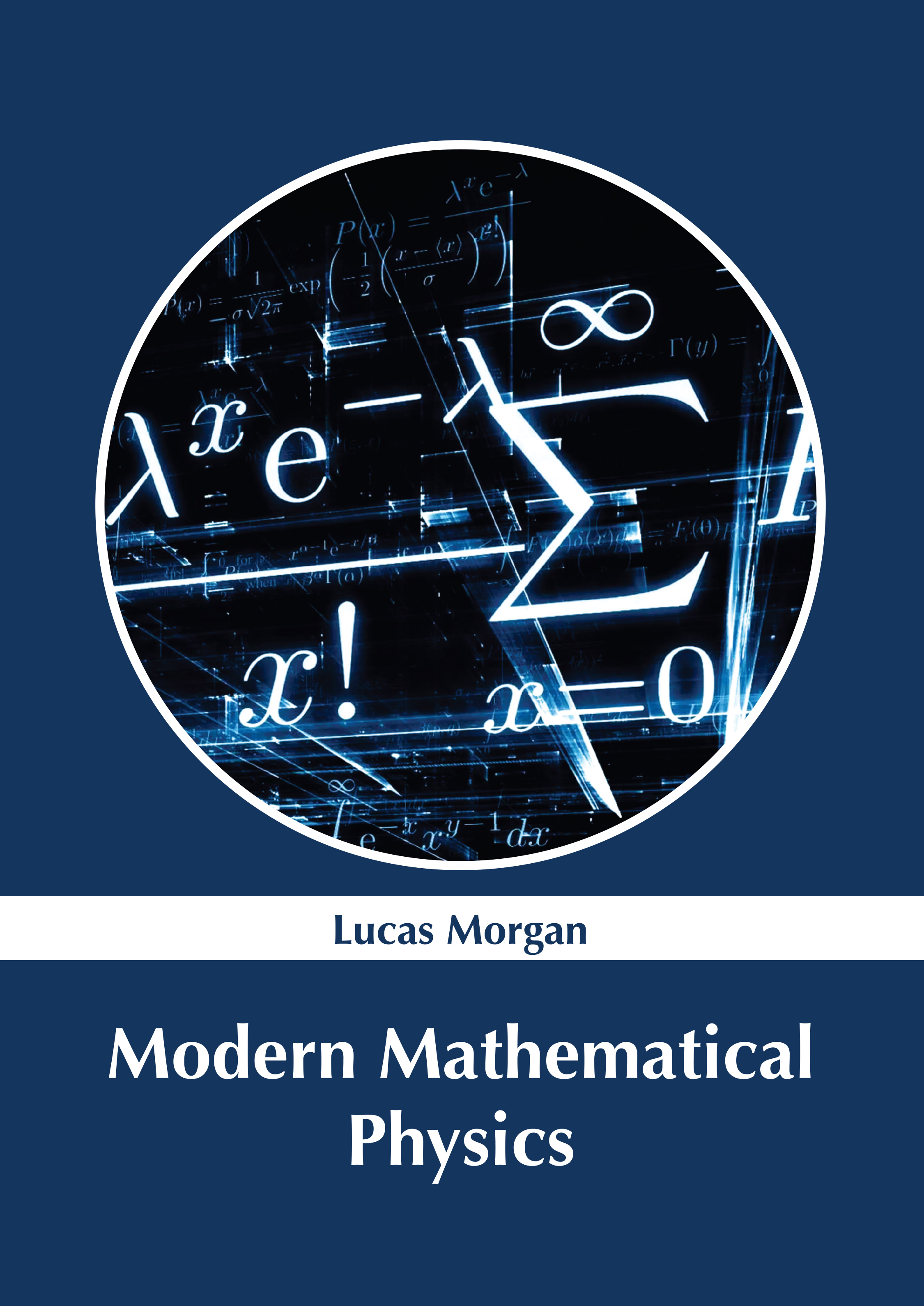BROWSE BY

#### Modern Mathematical Physics9781632387660

Author

Lucas Morgan

##### Publisher

NY Research Press

English

2021

##### Category

Physics - Physics

\$ 152.95

##### Description

Mathematical physics is a branch of physics that is concerned with the application of mathematical concepts to problems in physics. It focuses on solving such problems by formulating a mathematically rigorous framework. It is characterized by the blending of mathematical and physics theoretical aspects. Mathematical physics primarily expands and elucidates physical theories. There are several distinct branches of mathematical physics. These are partial differential equations, classical mechanics, quantum theory, relativity and quantum relativistic theories, and statistical mechanics. This book attempts to understand the multiple branches that fall under the discipline of mathematical physics and how such concepts have practical applications. It strives to provide a fair idea about this discipline and to help develop a better understanding of the latest advances within this field. This book includes contributions of experts and scientists which will provide innovative insights into mathematical physics.